mBuyy Website requires Javascript to be enabled!

# Beijing Normal University Bankou arithmetic problem card Grade 3 Grade 3 Second volume math arithmetic problem card + word problem card + vertical arithmetic card total 3 this arithmetic mental arithmetic application problem every day practice arithmetic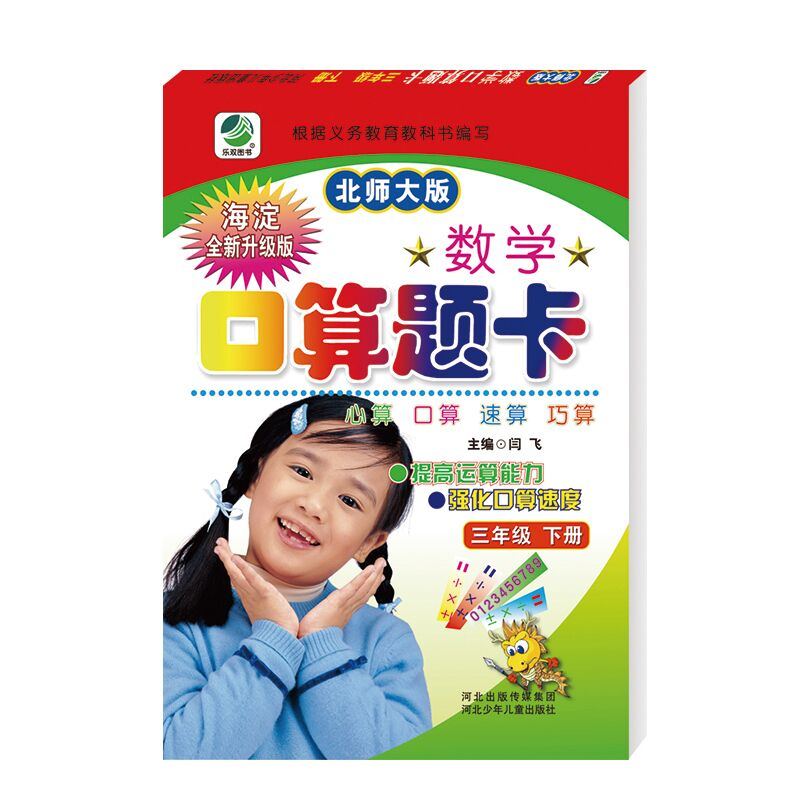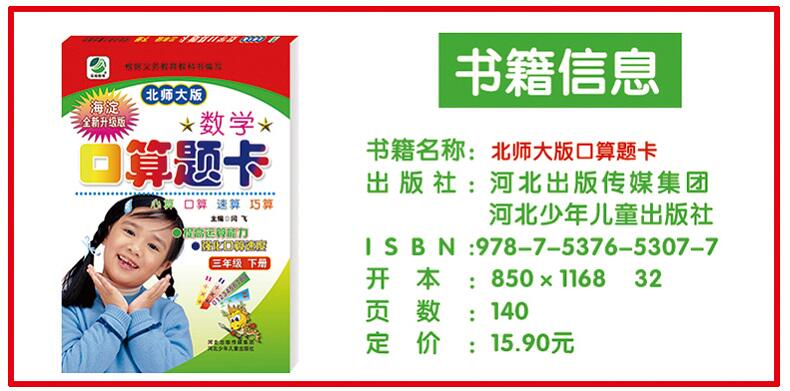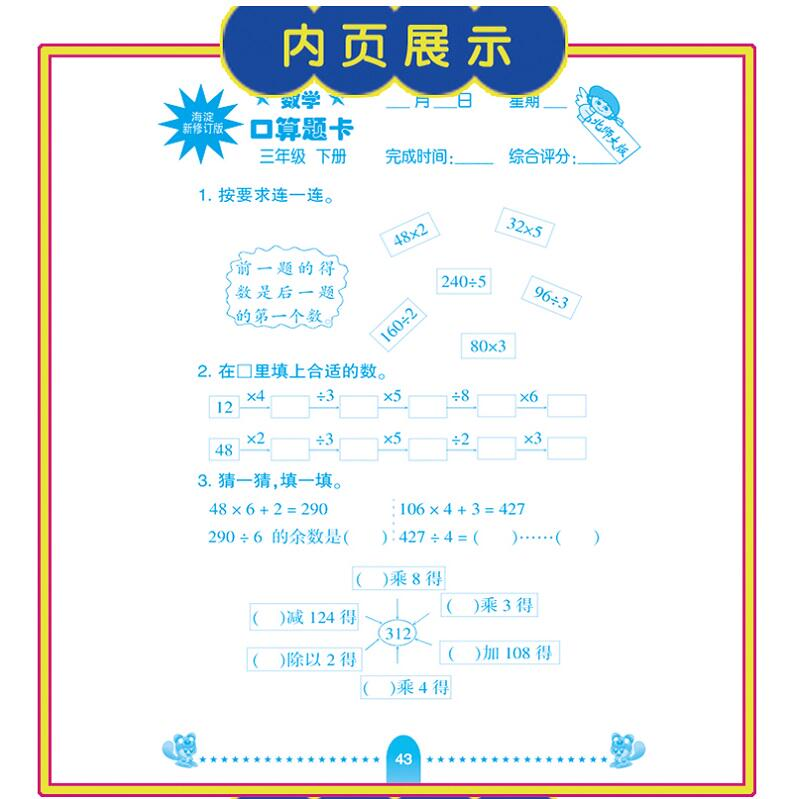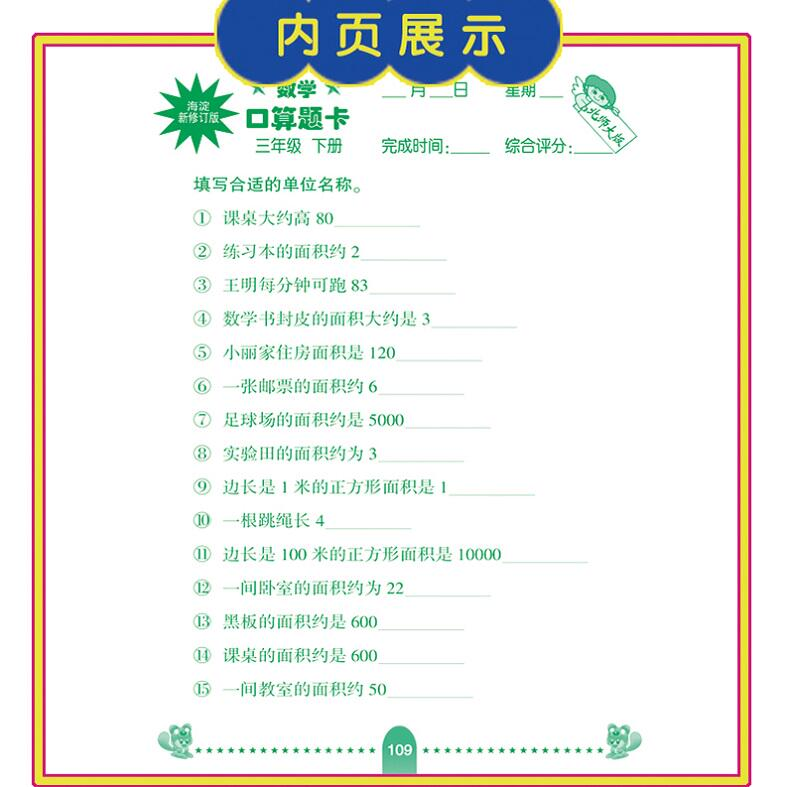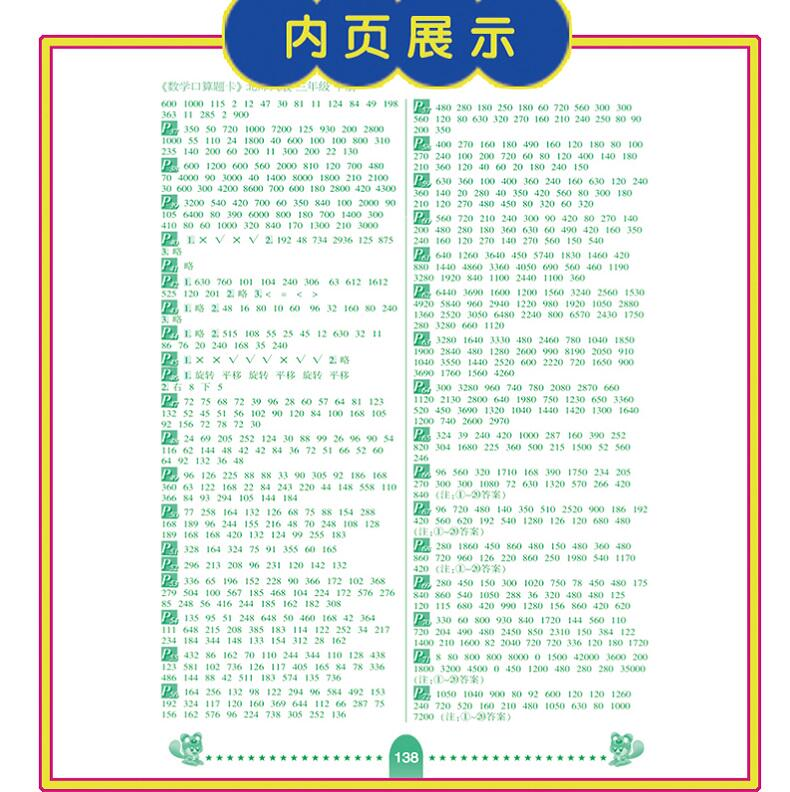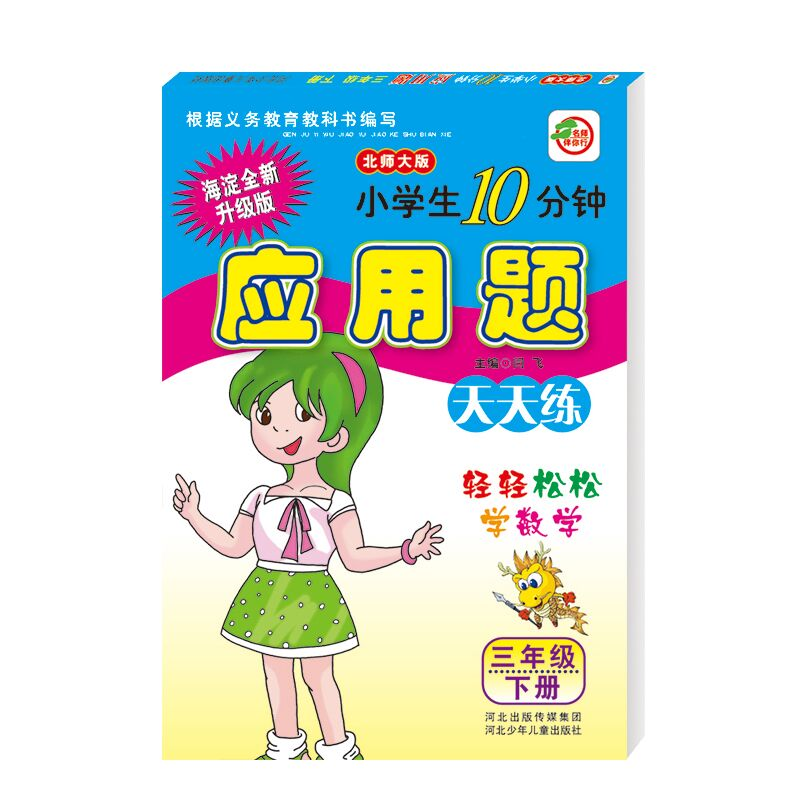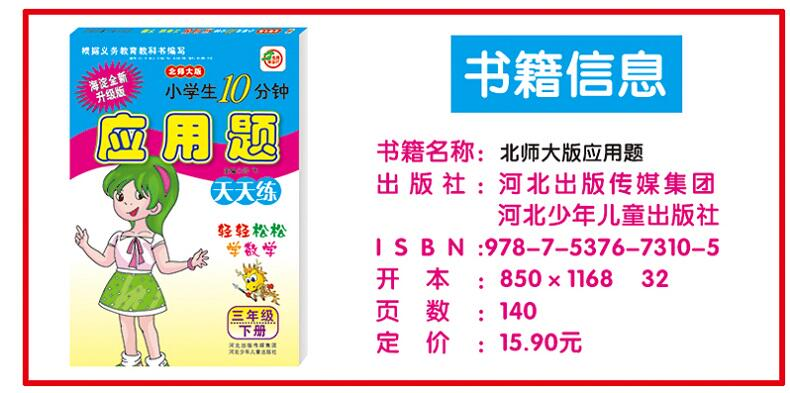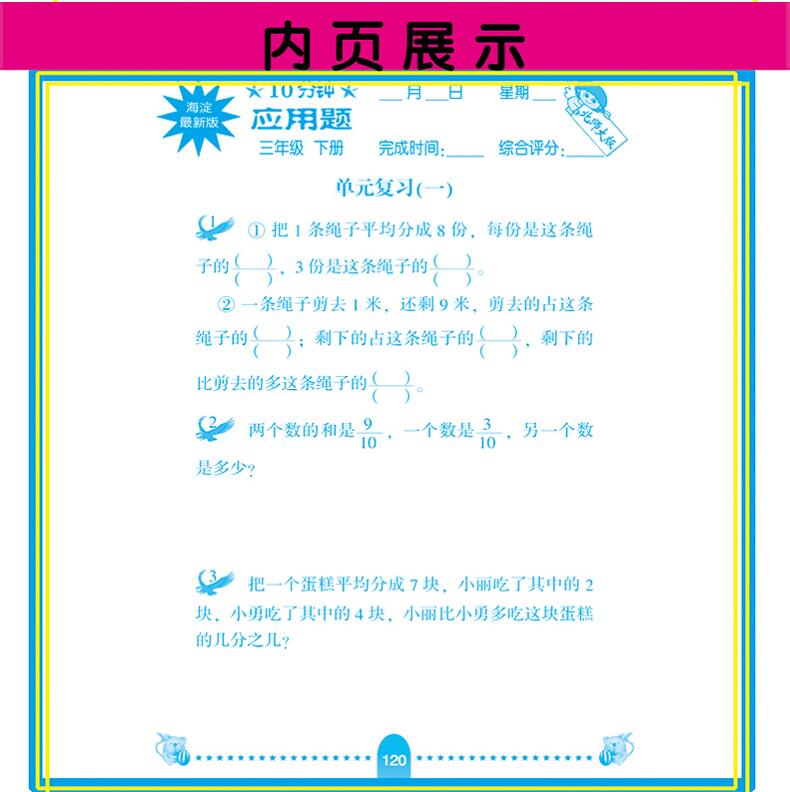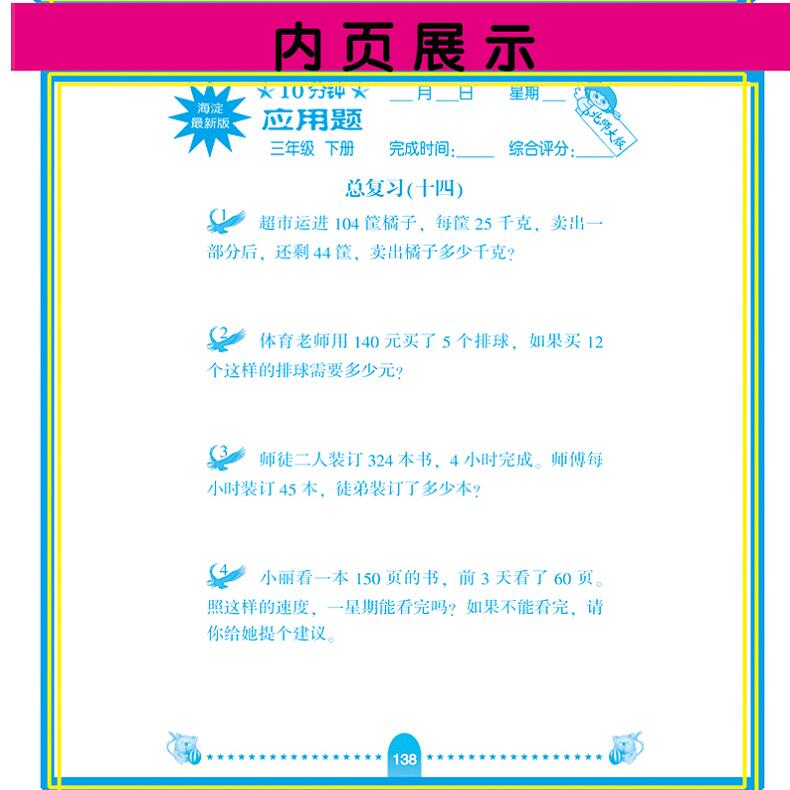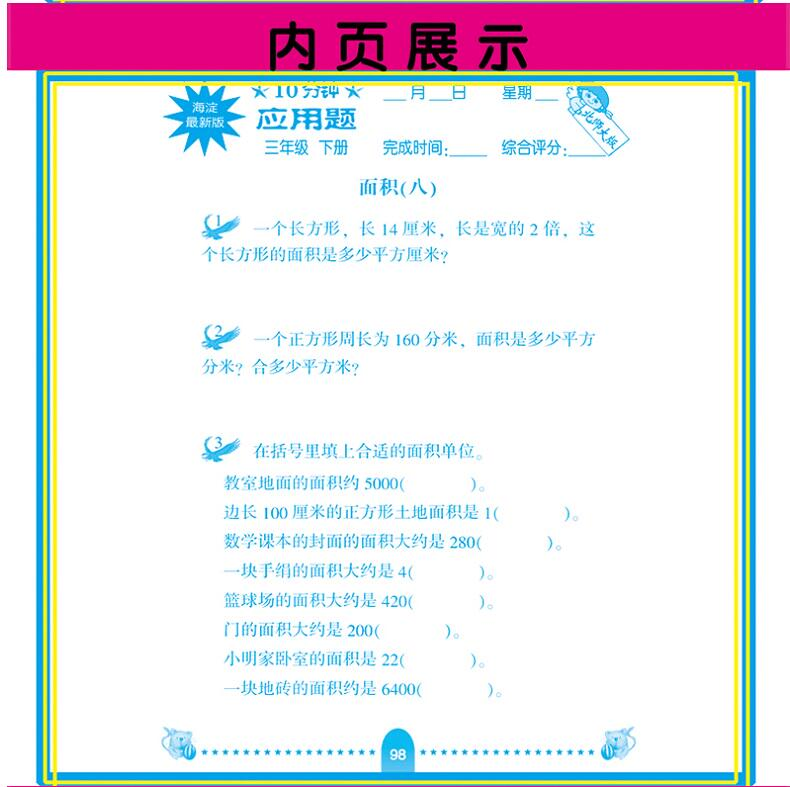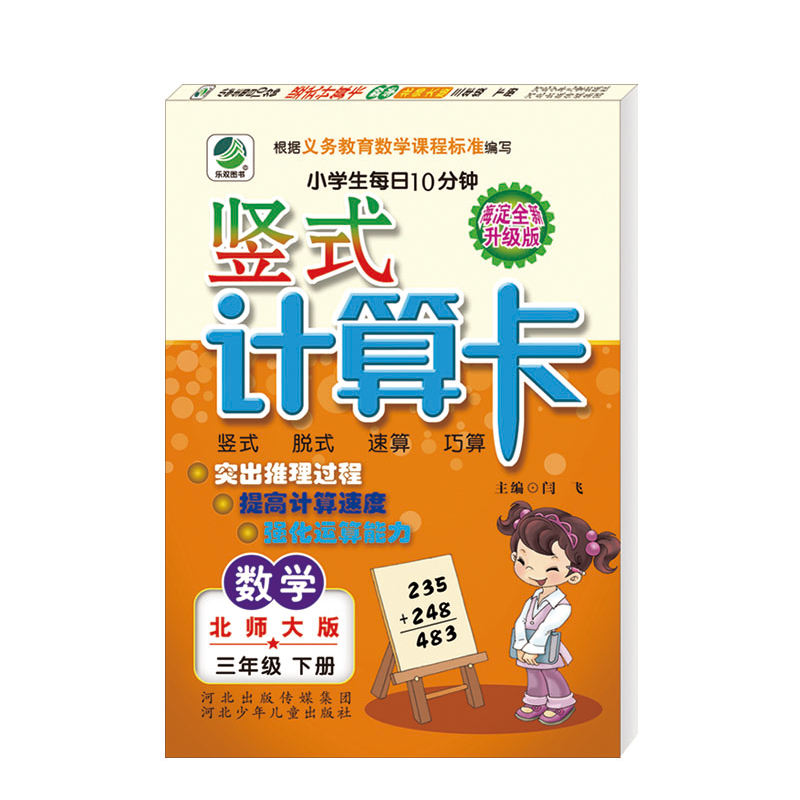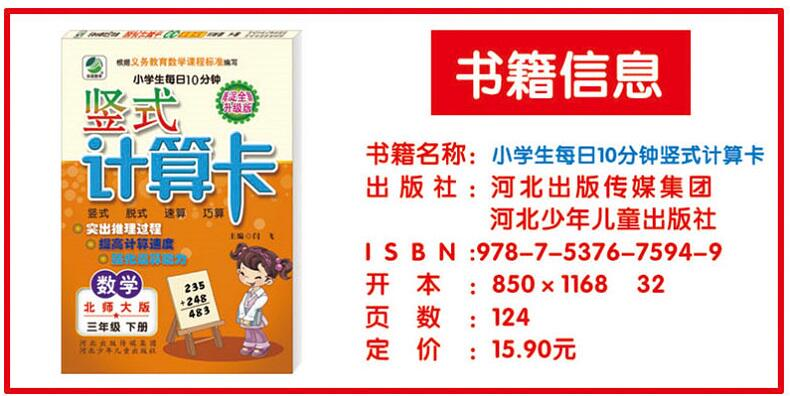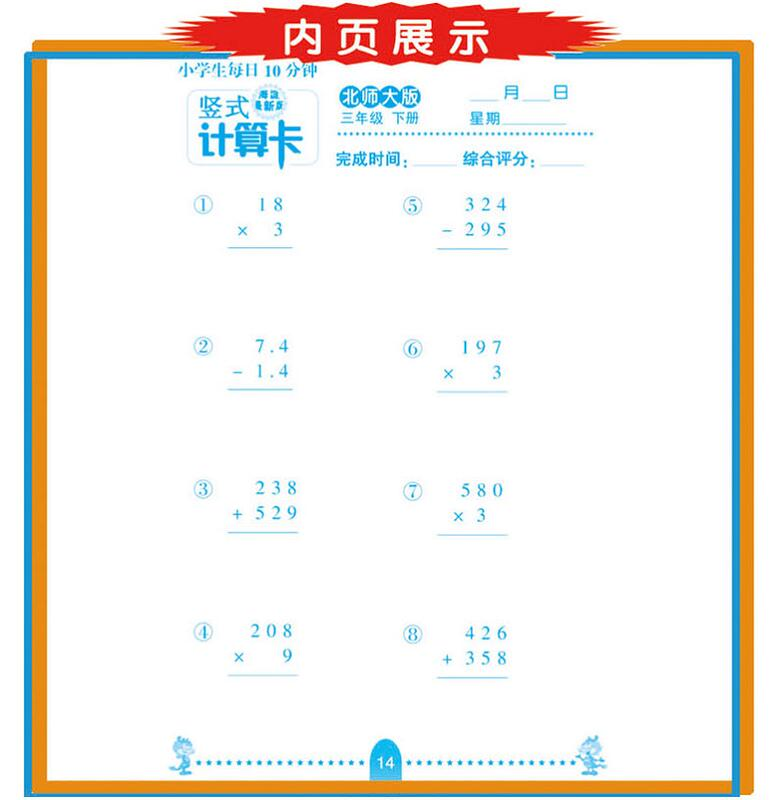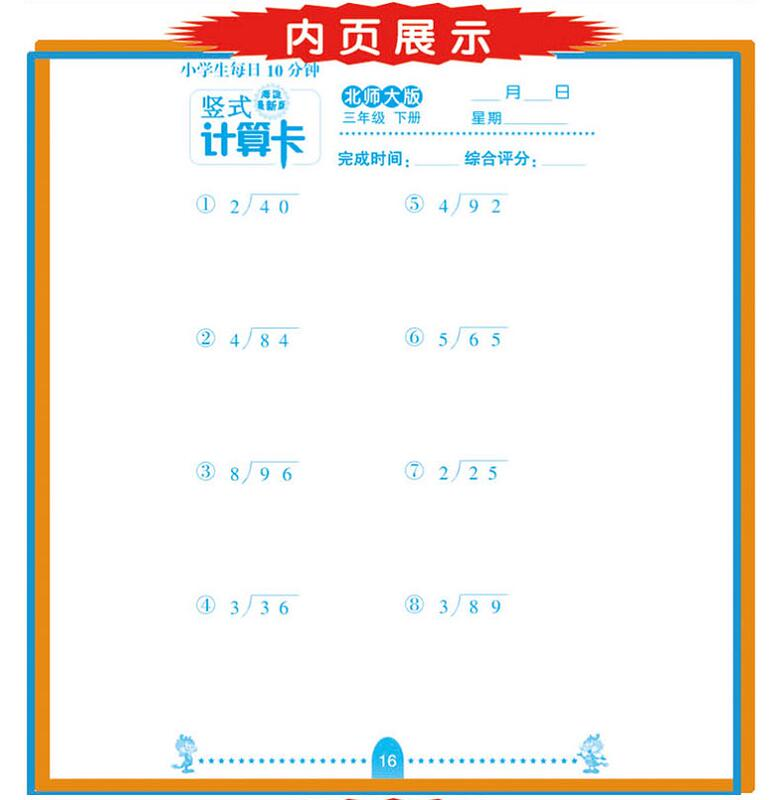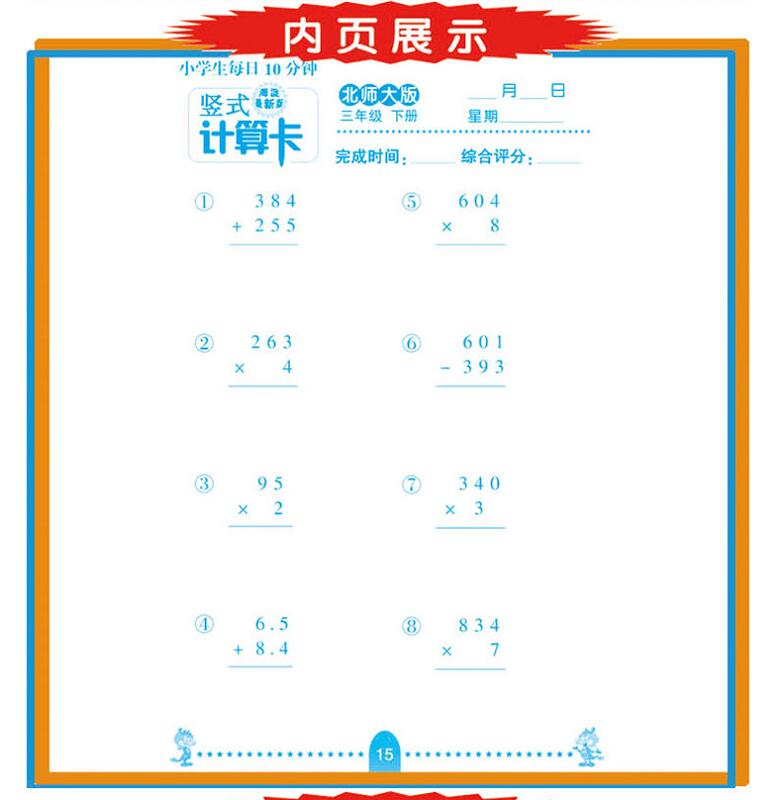Specifications ISBN number 9787537675949 Title 竖式计算卡 Author no Pricing 12.90 yuan Whether the suit no format 32 open Publisher Name Hebei Children's Publishing House

# Beijing Normal University Bankou arithmetic problem card Grade 3 Grade 3 Second volume math arithmetic problem card + word problem card + vertical arithmetic card total 3 this arithmetic mental arithmetic application problem every day practice arithmetic

• 3,444 Ks
• Ex Tax: 3,444 Ks